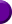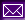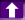The team Projects Articles FeaturesThe list of the winners of the contest which ended 04/18/99:

Place Name Score Sum
1. Clem Vasseur & Gregory Apou 137 137
2. Jeff Hellrung, Macross Software 139 139
3. Ian Collier 143 143

This ZIP contains all the solutions sent in. Be aware of the fact that some may not work! These were the problems to be solved:

Optimization: Size

Unless otherwise specified, all strings are Z-strings.

 Problem

Evaluate an arithmetic expression

 Input: HL ® expression Output: If the expression is valid, the result should be stored in HL, and the carry flag should be cleared. If the expression is not valid (see below), the carry flag should be set, and A should be set to an error code: 1 = invalid expression, 2 = divison by zero. You don't have to set A to anything special if the expression is valid. Special: You may use 8 byte of temporary storage for scalars. You don't have to worry about the stack space not being big enough!

In this problem, an arithmetic expression (from now on referred to as expr) is any of the following...

• An integer n, where -32767 <=n <= 32767
• -n (unary minus)
• -(expr) (unary minus)
• expr+expr
• expr-expr
• expr*expr
• expr/expr (integer divison)
• (expr)
Integer divison means that the result is truncated, and the remainder is discarded. For instance, 7/4=1, -23/5=-4.

Anything else is not an expression!! That means that no blanks are allowed! Furthermore, (), is not an expression.

Precedense

The precedense order is the same as in regular math, that is...

1. parenthesis
2. unary minus
3. multiplication and divison (from left to right)
4. addition and subtraction (from left to right)
You do not have to worry about precedense order of errors. That is, no test data will have both divison by zero and an invalid expression...

ASCII codes

The ASCII codes for the valid characters are...

• 0-9 has the ASCII codes 48-59 (dec) and 30-39 (hex)
• + has the ASCII code 43 (dec) and 2B (hex)
• - has the ASCII code 45 (dec) and 2D (hex)
• * has the ASCII code 42 (dec) and 2A (hex)
• / has the ASCII code 47 (dec) and 2F (hex)
• ( has the ASCII code 40 (dec) and 28 (hex)
• ) has the ASCII code 41 (dec) and 29 (hex)
Note that unary minus and binary minus uses the same character!! This is usually not the case in calculators, math programs etc. This shouldn't cause any coding problems as, for instance, --5 is not a valid expression (but -(-5) is).

Invalidity

If an expression is invalid, the error can be...

• Invalid character (example: 5*x)
• End of parenthesis missing (example: "5+(3+2")
• Unexpected end of parenthesis (example: "5*(2+3))")
• Number missing (example: "5-+2")
All these examples should return with the carry flag set and A should equal 1.
Another error is divison by zero (example: 3/(5-5)) where the function should return with carry set and A=2.

Important

• You may assume that all numbers will lie in the range -32767 - 32767 and that and partial result (if evaluated from left to right) will be in that range as well!
• Since multiplication and divison is a bit tricky, you don't have to write those routines! You will be provided with two external routines, Mult and Div (download the file multdiv.h). The arguments to those routines are explained in the file multdiv.h). Note that you must use these routines!! Just don't include them in the source file when submitting - the size of the multdiv.h will not be counted anyway.
• You do not have to worry about empty strings (that is, just a 0) because that special case is probably handled before calling the evaluation routine (if you were to implement the routine in a real program).
Examples

 Input: HL ® "5*(2-8)/3" Output: HL = -10, NC

 Input: HL ® "-(76/8)" Output: HL = -9, NC

 Input: HL ® "32767-32767-32767" Output: HL = -32767, NC

 Input: HL ® "2*(((100--100))+((5--5)))*2 Output: HL = 840, NCPage last updated on Sun Apr 25 23:57:52 1999